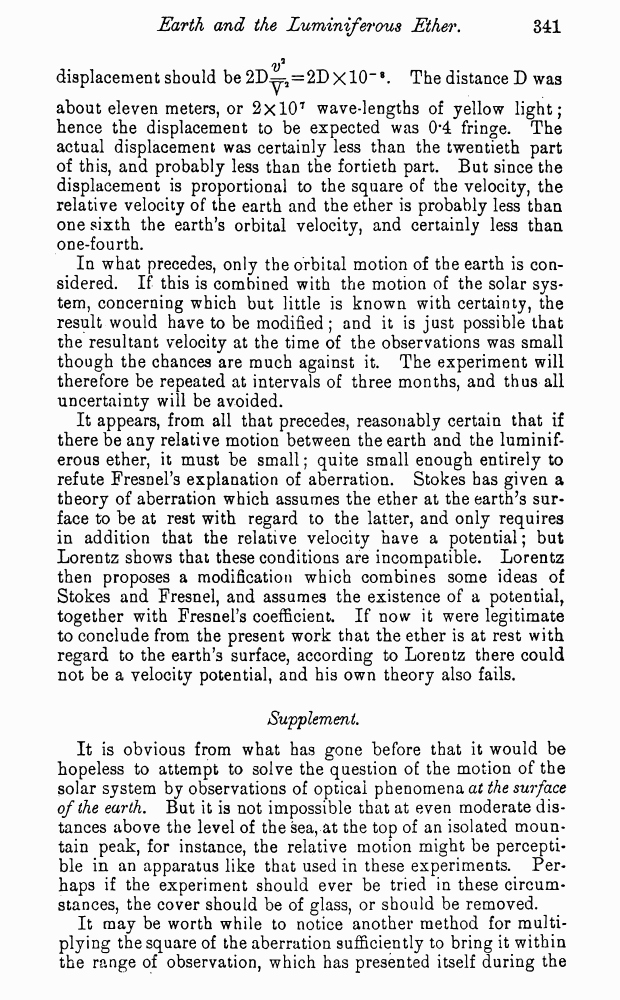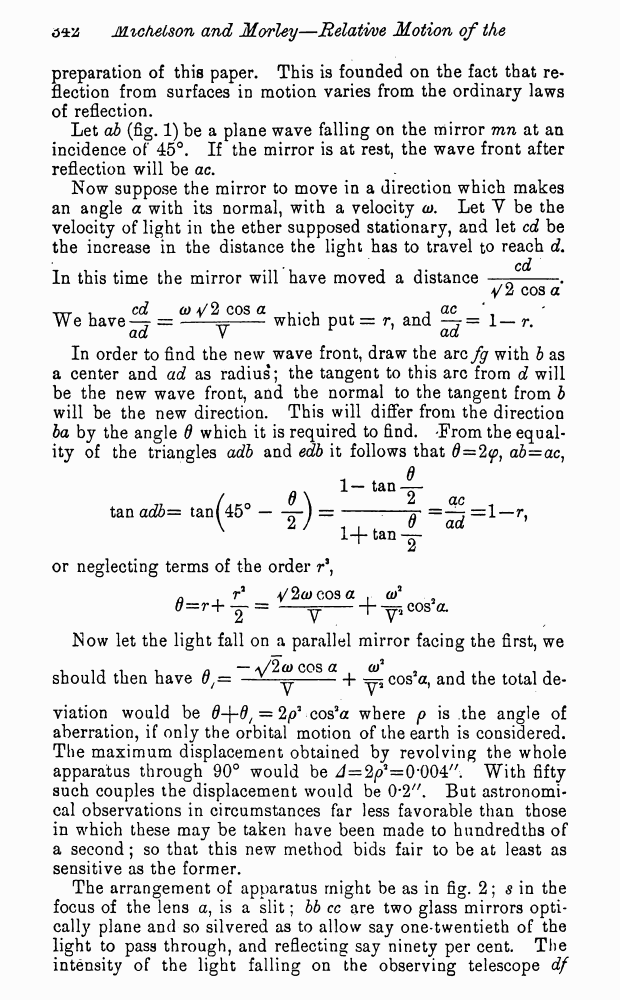# A. Michelson and E. Morley. On the Relative Motion of the Earth and the Luminiferous Ether. // American Journal of Science - Third series - Vol. XXXIV, No. 203. - Nov. 1887.

¬ Ìý˜ýÎÓ   ƒÛ„ËÂ ÙÓÏýÚ˚   <<<     —ÚýÌËˆý 341   >>>333  334  335  336  337  338  339  340  341 342  343  344  345 V displacement should be 2D^ra=2DxlO_Æ. The distance D was about eleven meters, or 2xl07 wave-lengths of yellow light; hence the displacement to be expected was 0*4 fringe. The actual displacement was certainly less than the twentieth part of this, and probably less than the fortieth part. But since the displacement is proportional to the square of the velocity, the relative velocity of the earth and the ether is probably less than one sixth the earthís orbital velocity, and certainly less than one-fourth. In what precedes, only the orbital motion of the earth is considered. If this is combined with the motion of the solar system, concerning which but little is known with certainty, the result would have to be modified ; and it is just possible that the resultant velocity at the time of the observations was small though the chances are much against it. The experiment will therefore be repeated at intervals of three months, and thus all uncertainty will be avoided. It appears, from all that precedes, reasonably certain that if there be any relative motion between the earth and the luminiferous ether, it must be small; quite small enough entirely to refute Fresnelís explanation of aberration. Stokes has given a theory of aberration which assumes the ether at the earthís surface to be at rest with regard to the latter, and only requires in addition that the relative velocity have a potential; but Lorentz shows that these conditions are incompatible. Lorentz then proposes a modification which combines some ideas of Stokes and Fresnel, and assumes the existence of a potential, together with Fresnelís coefficient. If now it were legitimate to conclude from the present work that the ether is at rest with regard to the earthís surface, according to Lorentz there could not be a velocity potential, and his own theory also fails. Supplement. It is obvious from what has gone before that it would be hopeless to attempt to solve the question of the motion of the solar system by observations of optical phenomena at the surface of the earth. But it is not impossible that at even moderate distances above the level of the sea, at the top of an isolated mountain peak, for instance, the relative motion might be perceptible in an apparatus like that used in these experiments. Perhaps if the experiment should ever be tried in these circumstances, the cover should be of glass, or should be removed. It may be worth while to notice another method for multiplying the square of the aberration sufficiently to bring it within the range of observation, which has presented itself during the preparation of this paper. This is founded on the fact that reflection from surfaces in motion varies from the ordinary laws of reflection. Let ab (fig. 1) be a plane wave falling on the mirror mn at an incidence of 45∞. If the mirror is at rest, the wave front after reflection will be ac. Now suppose the mirror to move in a direction which makes an angle a with its normal, with a velocity a). Let Y be the velocity of light in the ether supposed stationary, and let cd be the increase in the distance the light has to travel to reach d. cd In this time the mirror will have moved a distance ó--. 4/2 cos a Ñ . cd a) a/2 cos a . . , ^ , ac We haveó7 = ó-which put = r, and ó7= 1ó r. ad Y ad In order to find the new wave front, draw the arc fg with b as a center and ad as radius; the tangent to this arc from d will be the new wave front, and the normal to the tangent from b will be the new direction. This will differ from the direction ba by the angle d which it is required to find. 'From the equality of the triangles adb and edb it follows that d=2cosa . of . _ 0 = r+ y = -y--h y5C0Sa* Now let the light fall on a parallel mirror facing the first, we should then have 0f= cos a _j_ ^ COs2a, and the total de viation would be 0+0/ = cos3a where p is the angle of aberration, if only the orbital motion of the earth is considered. The maximum displacement obtained by revolving the whole apparatus through 90∞ would be J=2iai=0ë004//. With fifty such couples the displacement would be 0#2". But astronomical observations in circumstances far less favorable than those in which these may be taken have been made to hundredths of a second; so that this new method bids fair to be at least as sensitive as the former. The arrangement of apparatus might be as in fig. 2; s in the focus of the lens a, is a slit; bb cc are two glass mirrors optically plane and so silvered as to allow say one-twentieth of the light to pass through, and reflecting say ninety per cent. The intensity of the light falling on the observing telescope df